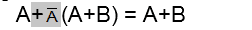# Boolean algebra

Hello,
Im trying to understand boolean algebra, I have to do the next problem using Boolean algebra and Identities for this one:So far this what I got:
A + /A (A+B) = A + B
A + /AA + /AB = A+B
A + A + /AB = A + B
A + A + B = A + B <-- I think A + A = A so ..
A + B = A + B <-- Im not sure what to do after this

hello luigihs!second, /AA is not A … what is it?